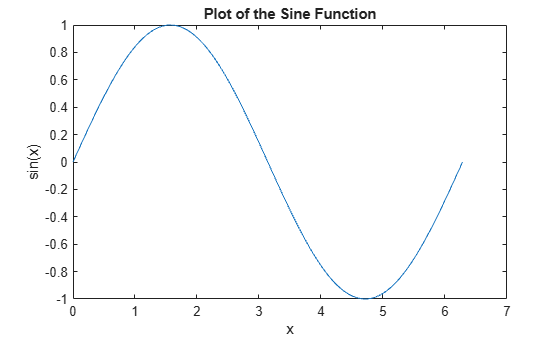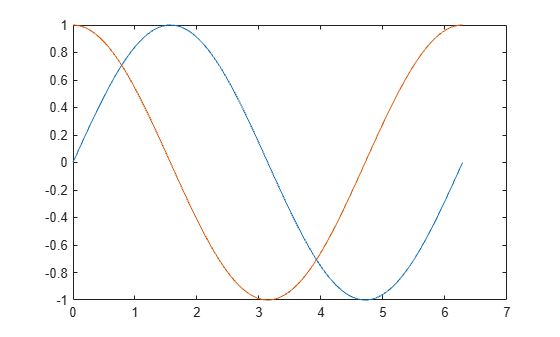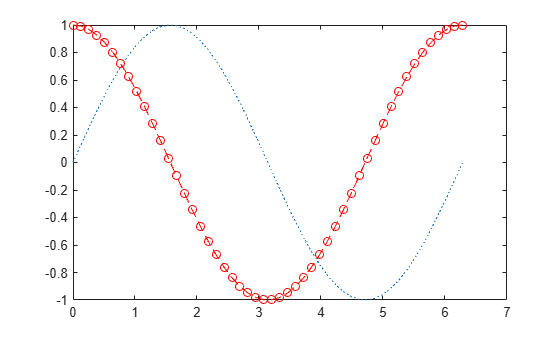# Create 2-D Line Plot

Create a simple line plot and label the axes. Customize the appearance of plotted lines by changing the line color, the line style, and adding markers.

### Create Line Plot

Create a two-dimensional line plot using the `plot` function. For example, plot the value of the sine function from 0 to $2\pi$.

```x = linspace(0,2*pi,100); y = sin(x); plot(x,y)```

Label the axes and add a title.

```xlabel('x') ylabel('sin(x)') title('Plot of the Sine Function')```### Plot Multiple Lines

By default, MATLAB clears the figure before each plotting command. Use the `figure` command to open a new figure window. You can plot multiple lines using the `hold on` command. Until you use `hold off` or close the window, all plots appear in the current figure window.

```figure x = linspace(0,2*pi,100); y = sin(x); plot(x,y) hold on y2 = cos(x); plot(x,y2) hold off```### Change Line Appearance

You can change the line color, line style, or add markers by including an optional line specification when calling the `plot` function. For example:

• `':'` plots a dotted line.

• `'g:'` plots a green, dotted line.

• `'g:*'` plots a green, dotted line with star markers.

• `'*'` plots star markers with no line.

The symbols can appear in any order. You do not need to specify all three characteristics (line color, style, and marker). For more information about the different style options, see the `plot` function page.

For example, plot a dotted line. Add a second plot that uses a dashed, red line with circle markers.

```x = linspace(0,2*pi,50); y = sin(x); plot(x,y,':') hold on y2 = cos(x); plot(x,y2,'--ro') hold off```Plot only the data points by omitting the line style option from the line specification.

```x = linspace(0,2*pi,25); y = sin(x); plot(x,y,'o')```### Change Line Object Properties

You also can customize the appearance of the plot by changing properties of the `Line` object used to create the plot.

Create a line plot. Assign the `Line` object created to the variable `ln`. The display shows commonly used properties, such as `Color`, `LineStyle`, and `LineWidth`.

```x = linspace(0,2*pi,25); y = sin(x); ln = plot(x,y)```
```ln = Line with properties: Color: [0 0.4470 0.7410] LineStyle: '-' LineWidth: 0.5000 Marker: 'none' MarkerSize: 6 MarkerFaceColor: 'none' XData: [0 0.2618 0.5236 0.7854 1.0472 1.3090 1.5708 1.8326 2.0944 2.3562 2.6180 2.8798 3.1416 3.4034 3.6652 3.9270 4.1888 4.4506 4.7124 4.9742 5.2360 5.4978 5.7596 6.0214 6.2832] YData: [0 0.2588 0.5000 0.7071 0.8660 0.9659 1 0.9659 0.8660 0.7071 0.5000 0.2588 1.2246e-16 -0.2588 -0.5000 -0.7071 -0.8660 -0.9659 -1 -0.9659 -0.8660 -0.7071 -0.5000 -0.2588 -2.4493e-16] Show all properties ```

To access individual properties, use dot notation. For example, change the line width to 2 points and set the line color to an RGB triplet color value, in this case `[0 0.5 0.5]`. Add blue, circle markers.

```ln.LineWidth = 2; ln.Color = [0 0.5 0.5]; ln.Marker = 'o'; ln.MarkerEdgeColor = 'b';```